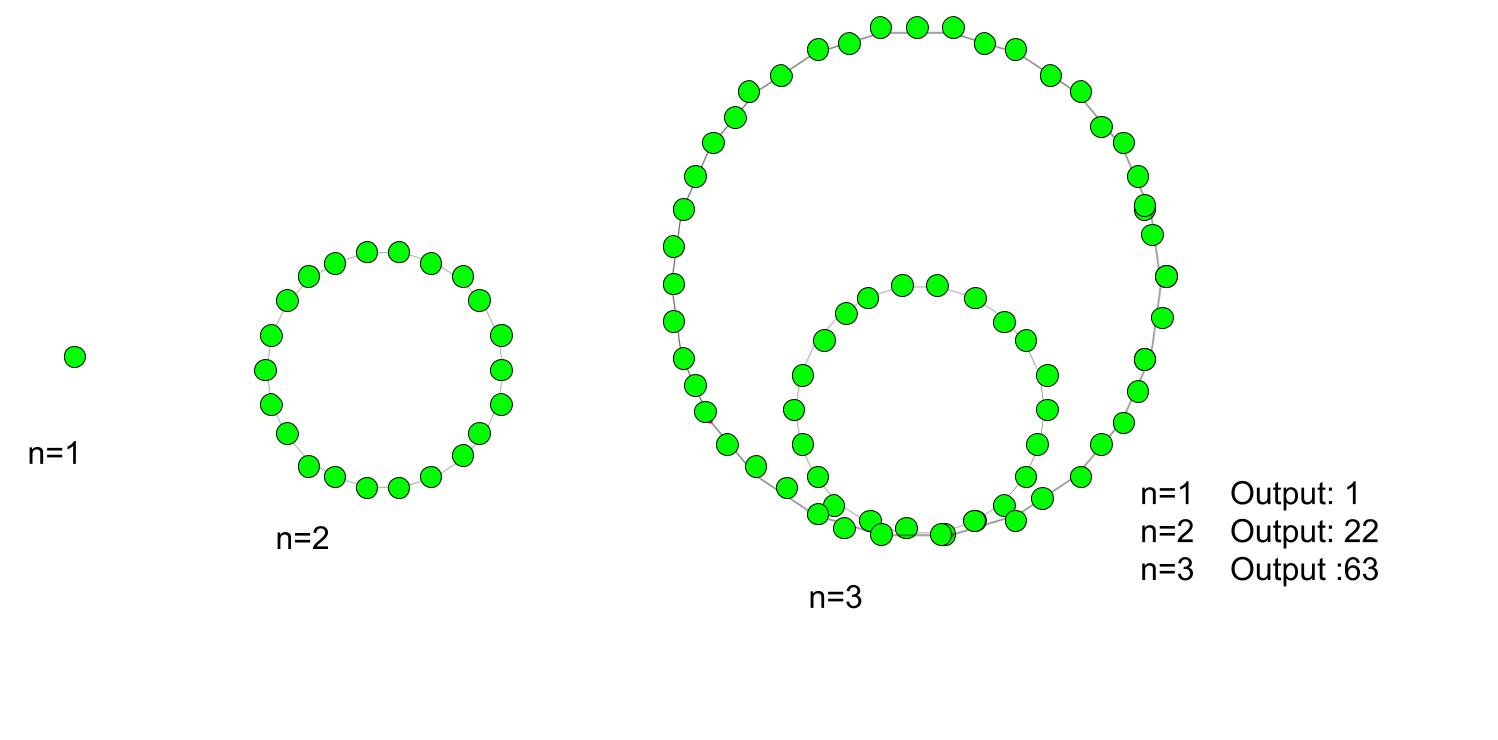# Icosidigonal number

• Last Updated : 20 May, 2022

Given a number n, the task is to find the nth Icosidigonal number (Isdn).
The polygon has the many gons, depends on their gonal number series. In mathematics, there is a number of gonal numbers and the icosidigonal number is one of them and these numbers have 22 -sided polygon (icosidigon). An icosidigonal number belong to the class of figurative number. They have one common dots points and other dots pattern is arranged in an n-th nested icosidigon pattern.
Examples :

Input : 2
Output :22
Input :6
Output :306Formula for nth Icosidigonal number:## C++

 // C++ program to find nth Icosidigonal// number#include using namespace std; // Function to calculate Icosidigonal numberint icosidigonal_num(long int n){    // Formula for finding    // nth Icosidigonal number    return (20 * n * n - 18 * n) / 2;} // Driver functionint main(){    long int n = 4;    cout << n << "th Icosidigonal number :" << icosidigonal_num(n);    cout << endl;    n = 8;    cout << n << "th Icosidigonal number:" << icosidigonal_num(n);    return 0;}

## C

 // C program to find nth Icosidigonal// number#include  // Function to calculate Icosidigonal numberint icosidigonal_num(long int n){       // Formula for finding    // nth Icosidigonal number    return (20 * n * n - 18 * n) / 2;} // Driver functionint main(){    long int n = 4;    printf("%ldth Icosidigonal number : %d\n",n,icosidigonal_num(n));     n = 8;    printf("%ldth Icosidigonal number : %d\n",n,icosidigonal_num(n));    return 0;} // This code is contributed by kothavvsaakash.

## Java

 // Java program to find nth// Icosidigonal numberimport java.io.*; class GFG{         // Function to calculate    // Icosidigonal number    static int icosidigonal_num(int n)    {             // Formula for finding    // nth Icosidigonal number         return (20 * n * n - 18 * n) / 2;    } // Driver Codepublic static void main (String[] args){     int n = 4;    System.out.print (n + "th Icosidigonal number :");    System.out.println (icosidigonal_num(n));     n = 8;    System.out.print (n + "th Icosidigonal number :");    System.out.println (icosidigonal_num(n));}} // This code is contributed by ajit

## Python 3

 # python 3 program to find# nth Icosidigonal number # Function to calculate# Icosidigonal numberdef icosidigonal_num(n) :         # Formula for finding    # nth Icosidigonal number    return (20 * n * n -            18 * n) // 2 # Driver Codeif __name__ == '__main__' :             n = 4    print(n,"th Icosidigonal " +                    "number : ",            icosidigonal_num(n))    n = 8    print(n,"th Icosidigonal " +                    "number : ",            icosidigonal_num(n))     # This code is contributed m_kit

## C#

 // C# program to find nth// Icosidigonal numberusing System; class GFG{         // Function to calculate    // Icosidigonal number    static int icosidigonal_num(int n)    {             // Formula for finding    // nth Icosidigonal number    return (20 * n * n -            18 * n) / 2;    } // Driver Codestatic public void Main (){int n = 4;Console.Write(n + "th Icosidigonal " +                          "number :");Console.WriteLine(icosidigonal_num(n)); n = 8;Console.Write (n + "th Icosidigonal "+                          "number :");Console.WriteLine(icosidigonal_num(n));}} // This code is contributed by ajit

## PHP

 

## Javascript

 

Output :

4th Icosidigonal number :124
8th Icosidigonal number:568

Time Complexity: O(1)
Auxiliary Space: O(1)
Reference: https://en.wikipedia.org/wiki/Polygonal_number

My Personal Notes arrow_drop_up